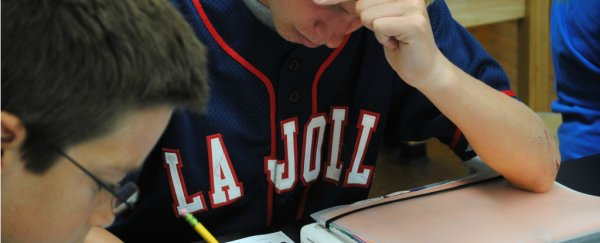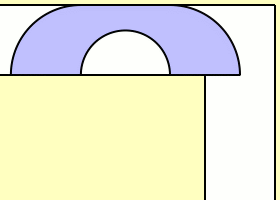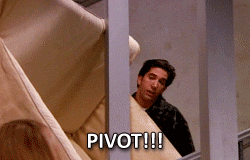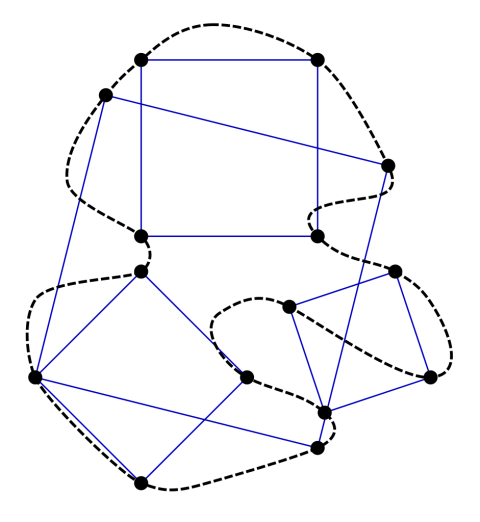# 6 Deceptively Simple Maths Problems That No One Can Solve

SCIENCEALERT STAFF
26 JANUARY 2018

We all know that maths is really hard. So hard, in fact, that there's literally a whole Wikipedia page dedicated to unsolved mathematical problems, despite some of the greatest minds in the world working on them around the clock.

But as Avery Thompson points out at Popular Mechanics, from the outset at least, some of these problems seem surprisingly simple - so simple, in fact, that anyone with some basic maths knowledge can understand them... including us. Unfortunately, it turns out that proving them is a little harder.

Inspired by Thompson's list, we've come up with our own list of deceptively simple maths problems to frustrate (and hopefully inspire) you.

The Twin Prime conjecture

Prime numbers are those magical unicorns that are only divisible by themselves and 1. As far as we know, there's an infinite number of primes, and mathematicians are working hard to constantly find the next largest prime number.

But is there an infinite amount of prime numbers pairs that differ by two, like 41 and 43? As primes get larger and larger, these twin primes are harder to find, but in theory, they should be infinite... the problem is no one's been able to prove that as yet.

The Moving Sofa ProblemClaudio Rocchini

This is something most of us have struggled with before - you're moving into a new apartment and trying to bring your old sofa along. But, of course, you have to maneuver it around a corner before you can get comfy on it in your living room.

Rather than giving up and just buying a beanbag, at this point, mathematicians want to know: what's the largest sofa you could possible fit around a 90 degree corner, regardless of shape, without it bending? (Although they're looking at the whole thing from a two-dimensional perspective.)

Thompson explains:

"The largest area that can fit around a corner is called - I kid you not - the sofa constant.

Nobody knows for sure how big it is, but we have some pretty big sofas that do work, so we know it has to be at least as big as them. We also have some sofas that don't work, so it has to be smaller than those. All together, we know the sofa constant has to be between 2.2195 and 2.8284."

We bet Ross from friends wishes someone had told him that.Friends/NBC

The Collatz conjectureXKCD

The Collatz conjecture is one of the most famous unsolved mathematical problems, because it's so simple, you can explain it to a primary-school-aged kid, and they'll probably be intrigued enough to try and find the answer for themselves.

So here's how it goes: pick a number, any number.

If it's even, divide it by 2. If it's odd, multiply it by 3 and add 1. Now repeat those steps again with your new number. Eventually, if you keep going, you'll eventually end up at 1 every single time (try it for yourself, we'll wait).

As simple as it sounds, it actually works. But the problem is that even though mathematicians have shown this is the case with millions of numbers, they haven't found any numbers out there that won't stick to the rules.

"It's possible that there's some really big number that goes to infinity instead, or maybe a number that gets stuck in a loop and never reaches 1," explains Thompson. "But no one has ever been able to prove that for certain."

The Beal conjecture

The Beal conjecture basically goes like this...

If A+ B= Cz

And A, B, C, x, y, and z are all positive integers (whole numbers greater than 0), then A, B, and C should all have a common prime factor.

A common prime factor means that each of the numbers needs to be divisible by the same prime number. So 15, 10, and 5 all have a common prime factor of 5 (they're all divisible by the prime number 5).

So far, so simple, and it looks like something you would have solved in high school algebra.

But here's the problem. Mathematicians haven't ever been able to solve the Beale conjecture, with x, y, and z all being greater than 2.

For example, let's use our numbers with the common prime factor of 5 from before....

51 + 101 = 151

but

52 + 102 ≠ 152

There's currently a US\$1 million prize on offer for anyone who can offer a peer-reviewed proof of this conjecture... so get calculating.

The Inscribed Square problemClaudio Rocchini

This one requires a little drawing. On a piece of paper, draw a loop - it doesn't have to be any set shape, just a closed loop that doesn't cross itself.

According to the inscribed square hypothesis, inside that loop, you should be able to draw a square that has all four corners touching the loop, just like in the diagram above.

Sounds simple... but mathematically speaking, there are a whole lot of possible loop shapes out there - and it's currently impossible to say whether a square will be able to touch all of them.

"This has already been solved for a number of other shapes, such as triangles and rectangles," writes Thompson, "But squares are tricky, and so far a formal proof has eluded mathematicians."

Goldbach's conjecture

Similar to the Twin Prime conjecture, Goldbach's conjecture is another famous and seemingly simple question about primes. It goes like this: is every even number greater than 2 the sum of two primes?

It sounds obvious that the answer would be yes, after all, 3 + 1 = 4, 5 + 1 = 6 and so on. At least, that was the original conjecture by German mathematician Christian Goldbach back in 1742.

Since then, we no longer follow the convention of seeing 1 as a prime, but the 'strong' version of Goldbach's conjecture lives on: all positive even integers larger than 4 can be expressed as the sum of two primes.

And yet, despite centuries of attempts, until now no one's been able to prove that this will always be the case. There was even a prize advertised for this in the early 2000s, but it went unclaimed.

The reality is that, as we continue to calculate larger and larger numbers, we may eventually find one that isn't the sum of two primes... or ones that defy all the rules and logic we have so far. And you can be sure mathematicians aren't going to stop looking until they find it.

Editor's note (19 May 2021): An earlier version of this article cited an incorrect example for Goldbach's conjecture. This has been clarified to explain how the conjecture has changed since its inception.